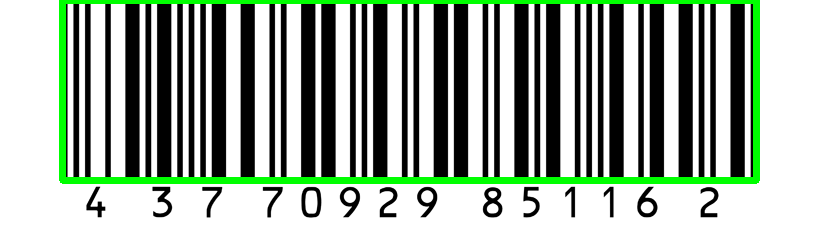# How to Make a Barcode Reader in Python

Learn how to make a barcode scanner that decodes barcodes and draw them in the image using pyzbar and OpenCV libraries in Python
5 min read · Updated jul 2022 · Computer Vision · Python for Multimedia

Disclosure: This post may contain affiliate links, meaning when you click the links and make a purchase, we receive a commission.

A barcode is a method of representing data in a visual and machine-readable form, it consists of bars and spaces. Today, we see barcodes everywhere, especially in products in supermarkets.

Barcodes can be read by an optical barcode scanner, but in this tutorial, we will make a script in Python that is able to read and decode barcodes, as well as a drawing where they're located in a given image.

To get started, we need to install few libraries:

``pip3 install pyzbar opencv-python``

Once you have these installed, open up a new Python file and import them:

``````from pyzbar import pyzbar
import cv2``````

I have few images to test with, you can use any image you want from the internet or your own disk, but you can get my test images in this directory.

I have wrapped every functionality into a function, the first function we gonna discuss is the following:

``````def decode(image):
# decodes all barcodes from an image
decoded_objects = pyzbar.decode(image)
for obj in decoded_objects:
# draw the barcode
print("detected barcode:", obj)
image = draw_barcode(obj, image)
# print barcode type & data
print("Type:", obj.type)
print("Data:", obj.data)
print()

return image``````

`decode()` function takes an image as a numpy array, and uses `pyzbar.decode()` that is responsible for decoding all barcodes from a single image and returns a bunch of useful information about each barcode detected.

We then iterate over all detected barcodes and draw a rectangle around the barcode and prints the type and the data of the barcode.

To make things clear, the following is how each `obj` looked like if we print it:

``Decoded(data=b'43770929851162', type='I25', rect=Rect(left=62, top=0, width=694, height=180), polygon=[Point(x=62, y=1), Point(x=62, y=179), Point(x=756, y=180), Point(x=756, y=0)])``

So `pyzbar.decode()` function returns the data containing the barcode, the type of barcode, as well as the location points as a rectangle and a polygon.

This brings us to the next function that we used, `draw_barcode()`:

``````def draw_barcode(decoded, image):
# n_points = len(decoded.polygon)
# for i in range(n_points):
#     image = cv2.line(image, decoded.polygon[i], decoded.polygon[(i+1) % n_points], color=(0, 255, 0), thickness=5)
# uncomment above and comment below if you want to draw a polygon and not a rectangle
image = cv2.rectangle(image, (decoded.rect.left, decoded.rect.top),
(decoded.rect.left + decoded.rect.width, decoded.rect.top + decoded.rect.height),
color=(0, 255, 0),
thickness=5)
return image``````

This function takes the decoded object we just saw, and the image itself, it draws a rectangle around the barcode using `cv2.rectangle()` function, or you can uncomment the other version of the function; drawing the polygon using `cv2.line()` function, the choice is yours. I preferred the rectangle version.

Finally, it returns the image that contains the drawn barcodes. Now let's use these functions for our example images:

``````if __name__ == "__main__":
from glob import glob

barcodes = glob("barcode*.png")
for barcode_file in barcodes:
# load the image to opencv
# decode detected barcodes & get the image
# that is drawn
img = decode(img)
# show the image
cv2.imshow("img", img)
cv2.waitKey(0)``````

In my current directory, I have barcode1.png, barcode2.png, and barcode3.png, which are all example images of a scanned barcode, I used glob so I can get all these images as a list and iterate over them.

On each file, we load it using `cv2.imread()` function, and use the previously discussed `decode()` function to decode the barcodes and then we show the actual image.

Note that this will also detect QR codes, and that's fine, but for more accurate results, I suggest you check the dedicated tutorial for detecting and generating qr codes in Python.

When I run the script, it shows each image and prints the type and data of it, press any key and you'll get the next image, here is my output:

``````detected barcode: Decoded(data=b'0036000291452', type='EAN13', rect=Rect(left=124, top=58, width=965, height=812), polygon=[Point(x=124, y=59), Point(x=124, y=869), Point(x=621, y=870), Point(x=1089, y=870), Point(x=1089, y=58)])
Type: EAN13
Data: b'0036000291452'

detected barcode: Decoded(data=b'Wikipedia', type='CODE128', rect=Rect(left=593, top=4, width=0, height=294), polygon=[Point(x=593, y=4), Point(x=593, y=298)])
Type: CODE128
Data: b'Wikipedia'

detected barcode: Decoded(data=b'43770929851162', type='I25', rect=Rect(left=62, top=0, width=694, height=180), polygon=[Point(x=62, y=1), Point(x=62, y=179), Point(x=756, y=180), Point(x=756, y=0)])
Type: I25
Data: b'43770929851162'``````

Here is the last image that is shown:## Conclusion

That is awesome, now you have a great tool to make your own barcode scanner in Python. I know you all want to read directly from the camera, as a result, I have prepared the code that reads from the camera and detects barcodes in a live manner, check it here!

You can also add some sort of a beep when each barcode is detected, just like in supermarkets, check the tutorial for playing sounds that may help you accomplish that.

For more detailed information, I invite you to check pyzbar documentation.

Finally, if you're a beginner and want to learn Python, I suggest you take the Python For Everybody Coursera course, in which you'll learn a lot about Python. You can also check our resources and courses page to see the Python resources I recommend on various topics!

Learn also: How to Generate and Read QR Code in Python.

Happy Coding ♥

Sharing is caring!

### Comment panel